HashTable 哈希表

## 1、什么是哈希表

这里我们首先借用一个leetcode上的一道题目来引入哈希表的概念。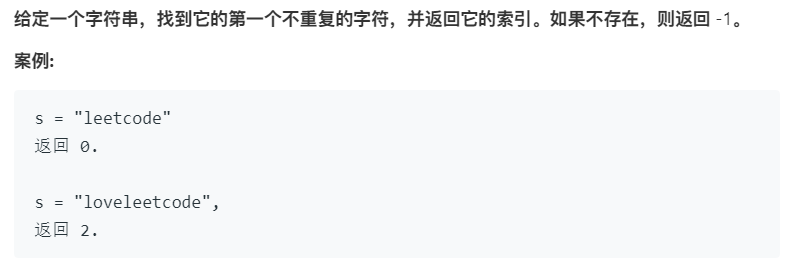我们知道解决这个问题可以使用一个我们之前的Map映射来解决这个问题，保存出现的每一个字符作为键值，出现的次数作为值，创建类似于 Map<Character, Integer> 这样的映射。

class Solution {
public int firstUniqChar(String s) {
Map<Character, Integer> map = new TreeMap<>();
for(int i = 0; i < s.length(); i++){
char c = s.charAt(i);
map.put(c, map.get(c) == null ? 1: map.get(c) + 1);
}
for(int i = 0; i < s.length(); i++)
if(map.get(s.charAt(i)) == 1)
return i;
return -1;
}
}


可以发现最后运行的效率特别低，所以可以对此进行更改底层实现。在题目的下方标注着：

所以说可以使用固定的数组来进行实现。数组大小为 26，数组索引的方式char - 'a'通过这样的方式来找到对应的字符。

class Solution {
public int firstUniqChar(String s) {
int[] code = new int;
for (int i = 0; i < s.length(); i++)
code[s.charAt(i) - 'a']++;
for (int i = 0; i < s.length(); i++)
if (code[s.charAt(i) - 'a'] == 1)
return i;
return -1;
}
}


使用数组（随机访问）实现起来的效率远大于Map的实现效率。char - 'a'就是哈希的哈希函数。哈希表就是将真正关心的内容存储到数组当中，时间复杂度就变成了O(1)级别。

哈希函数就是将“键”转换为“索引”。而且可以发现我们在哈希表上查找元素的时候时间复杂度为O（1）级别的。所以说我们最后实现的哈希表得时间复杂度为O(1)级别的。在哈希表上的操作难点也就在与如何解决哈希冲突问题上。

## 2、哈希函数的设计原则

1. 一致性：如果a=b，则hash(a) = hash(b)，反之不一定成立，对应哈希冲突
2. 高效性：计算速度快，简便
3. 均匀性：哈希值分布均匀

### 2.1、整型

• 小范围的正整数可以直接进行使用
• 小范围的负整数可以通过偏移进行归正

通常的方法是对其进行取模操作，只保留最后的几位，或者具有特征的几位。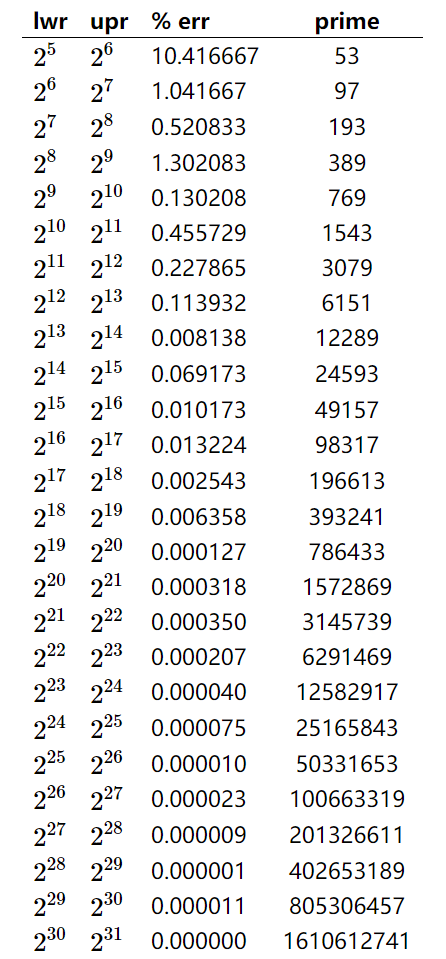• lwr：数据大小的下界
• upr：数据大小的上界 也就是数据大小位于（lwr - upr）之间
• err：错误率
• primer：模值的取值（素数）

### 2.2、浮点型

我们知道在我们的计算机当中，对于浮点数的存储也是采用二进制的形式，只不过计算机解析成了浮点数。下面以32位计算机为例。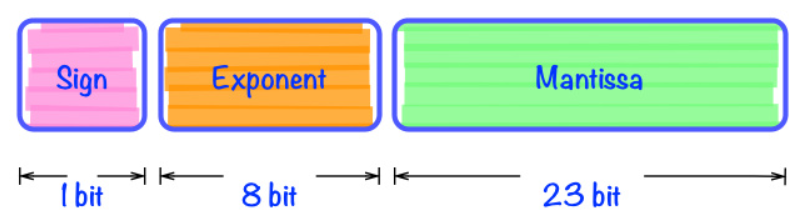### 2.3、字符串

这里字符串依然按照整型进行处理。我们知道整型有下面的变换公式：
$$2020 = 2 \times 103 + 0 \times 102 + 2 \times 101 + 2 \times 100$$

$$java = j \times 263 + a \times 262 + v \times 261 + a \times 260$$
这里我们默认只有小写字母，使用26进制，如果字符串中包含更加复杂的字符，例如大小写，标点符号等等。那么可以使用下面的形式：
$$Java = J \times I3 + a \times I2 + v \times I1 + a \times I0$$
I 表示进制，I的数值表示所代表类型的大小。对于字符串产生的这种大整型，我们可以按照2.1节那种，对大整型进行取模。
$$hash(Java) = (J \times I3 + a \times I2 + v \times I1 + a \times I0) % M$$

$$hash(Java) = (((J \times I + a)\times I + v) \times I + a) % M$$

但是上面的式子在不断进行乘法运算的时候，导致在取模之前的数值会变得十分巨大，所以对上面的式子继续进行化简。
$$hash(Java) = (((J %M \times I + a)%M\times I + v)%M \times I + a) % M$$

int hash = 0;
for(int i = 0; i < s.length; i++)
hash = (hash * I + s.charAt(i)) % M


### 2.3、Java 中的 hashCode()

在Java中的所有类都具有的hasCode()方法，因为hasCode方法在公共基类Object类中，类似于toString()方法。

当然可以重载hasCode的方法，这样我们在创建类似于HashSet这类底层使用哈希存储的容器类的时候就会调用自己的实现的hasCode方法。

class Dog{
private String name;
private int age;
private Date birthday;
public Dog(){
name = "WangCai";
age = 4;
birthday = new Date(2020, Calendar.JUNE, 16);
}

@Override
public int hashCode() {  //重载hashCode
return name.hashCode() + age + birthday.hashCode();
}
}


这样我们在调用 HashSet <Dog> set = new HashSet<>()，就会调用自己的hashCode()进行哈希存储。

## 3、哈希冲突的处理——链地址法

根据前面讲解的哈希表的哈希函数，需要对大整型进行取模的操作，其实最后的存储的数据大小就是模值。存储的方式就是采用和动态数组类似的方式，底层采用数组的方式存储。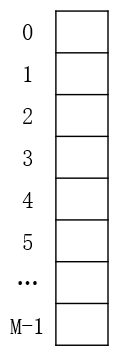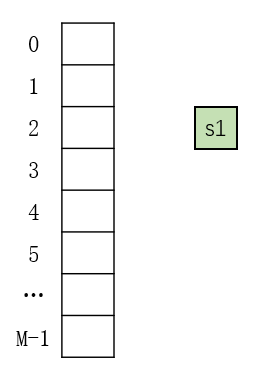index = ( hashCode(s1) & 0x7fffffff ) % M

在前面中说到 Java 中的 hasCode 可以返回负值，但是数组索引没有负值，所以需要将最前面的符号位设置为零。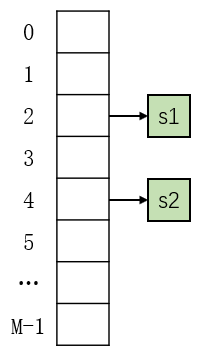当添加第三个元素（s3）的时候，假设获得的索引依然是 2 。这样就产生了哈希冲突。那么我们就需要将新添加的元素 s3 放到 s1 后面，类似于链表的形式。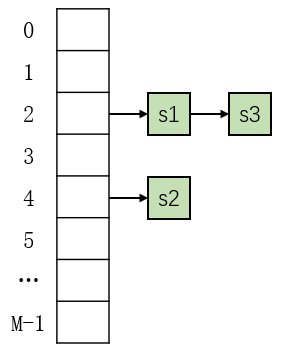当然前面的形式我们采用链表的形式，其实对于每一个索引位置我们可以索引一个树结构，也就降低了时间复杂度。类似于下面的形式。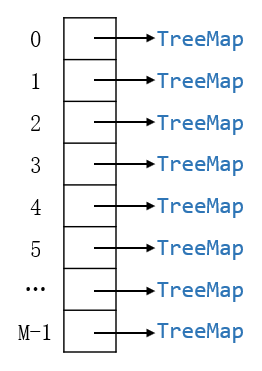实际上HashMap 的底层就是 TreeMap 数组；HashSet 的底层就是 TreeSet 数组；

## 4、HashTable的实现

这里我们以HashMap的实现为例，我们前面了解到HashMap的底层实现是TreeMap数组，所以我们的类中的变量就必须包含TreeMap数组。

### 4.1、初始化操作

• hashTable 数组，里面对应的类型为TreeMap。
• M：对应取模的值，也就是我们数组的大小
• size：用来存储用户数据的大小
public class HashTable<K, V> {

private TreeMap<K, V>[] hashTable;
private int M;
private int size;

public HashTable(int M) {
this.M = M;
size = 0;
hashTable = new TreeMap[M];
for (int i = 0; i < M; i++)
hashTable[i] = new TreeMap<>(); //对每一个TreeMap进行初始化
}

public HashTable(){
this(97);
}
}


### 4.2、哈希函数

哈希函数主要是通过获得key的hashCode()进行取模转换后来获得数组索引值。

private int hash(K key) {
return (key.hashCode() & 0x7FFFFFFF) % M;
}


### 4.3、增删改查操作

所有的基本操作都是首先通过hash(key)获得哈希值进而获得索引值。在通过索引值获得对应索引位置的TreeMap实例。然后对实例进行增删改查操作。

public void add(K key, V value) {
TreeMap<K, V>map = hashTable[hash(key)]; //获得 TreeMap
if (map.containsKey(key))
map.put(key, value);
else{
map.put(key, value);
size++;
}
}
}


public V remove(K key) {
TreeMap<K, V> map = hashTable[hash(key)];
if (map.containsKey(key)) {
size--;
return map.remove(key);
}
return null;
}


public void set(K key, V value) {
TreeMap<K, V> map = hashTable[hash(key)];
if (map.containsKey(key))
map.put(key, value);
}


public boolean contains(K key) {
TreeMap<K, V> map = hashTable[hash(key)];
return map.containsKey(key);
}

public V get(K key) {
return hashTable[hash(key)].get(key);
}


## 5、动态空间处理

最开始的时候我们说过我们在查找的元素时候时间复杂度为O(1)。但是前面的实现经过分析可以得出来，时间复杂度并不为O(1)级别。对于地址为链表的形式，时间复杂度为O(N/M)；对于地址为平衡树的形式，时间复杂度为O(log(N/M))。

所以说我们的实现结构还可以进行优化。优化的方式就是动态空间法，类似于之前的动态数组的实现，底层的数组大小是根据用户数据的大小进行动态改变的。

按照之前实现动态数组的方法，引入resize函数，触发resize函数的条件分别有以下两个条件：

• N\M >= upperTol:上界容忍度，触发扩容操作
• N\M <=lowwerTol:下届容忍度，触发缩容操作

### 5.1、初始化操作

• capacity: 容量，这是动态更改数组大小的值，全部为素数，取值来源于第2.1节整型。
• upperTol:上界容忍度
• lowerTol:下界容忍度
• capacityIndex:当前capacity的索引值，进而表示当前数组大小
• 其余变量不再做解释
public class HashTable<K, V> {  // K不具有可比较性

private final int[] capacity = {
53, 97, 193, 389, 769, 1543, 3079, 6151, 12289, 24593,
49157, 98317, 196613, 393241, 786433, 1572869, 3145739,
6291469,12582917, 25165843, 50331653, 100663319, 201326611,
402653189, 805306457, 1610612741
};

private static final int upperTol = 10;
private static final int lowerTol = 2;
private int capacityIndex = 0;
private TreeMap<K, V>[] hashTable;
private int M;
private int size;

public HashTable() {
this.M = capacity[capacityIndex];  //获取当前数组大小
size = 0;
hashTable = new TreeMap[M];
for (int i = 0; i < M; i++)
hashTable[i] = new TreeMap<>();
}
}


### 5.2、resize 更改容量操作

同动态数组相同，将原来的数组复制到一个新的容器大小的数组中。通过遍历就哈希表中的所有数据，重新添加到新的哈希表中。

private void resize(int newM) {
TreeMap<K, V>[] newHashTable = new TreeMap[newM];
for (int i = 0; i < newM; i++)
newHashTable[i] = new TreeMap<>();
int oldM = this.M;
this.M = newM; //必须进行更新，后面用到hash()建立在新的M上
for (int i = 0; i < oldM; i++) { //遍历哈希表中所有元素
TreeMap<K, V> map = hashTable[i];
for (K key : map.keySet())
newHashTable[hash(key)].put(key, map.get(key));
}
this.hashTable = newHashTable;
}


### 5.3、更新增删改查操作

由于导致数据变化的只有增删操作，对于更改和查询操作并不涉及，具体的更改只需要添加两行代码分别在增删操作上。

if (size >= upperTol * M && capacityIndex + 1 < capacity.length)
resize(capacity[++capacityIndex]);


if (size < lowerTol * M && capacityIndex >= 1)
resize(capacity[--capacityIndex]);


## 6、时间复杂度分析

### 6.1、未增加动态空间处理

我们假设数组大小为 M，用户存储的数组为 N。对应着每个地址所存储的元素个数 N / M（平均来说）。
|类型|时间复杂度|
|:---:|:---:|
|地址为链表|O(N/M)|
|地址为平衡树|O(log(N/M))|

### 6.2、增加动态空间处理

对于增删改查四种操作时间复杂度都是O(log(N/M))级别的。但是我们设置了upperTol和lowerTol。lowerTol <= N\M <= upperTol。两者都是自己设置的常数，所以说时间复杂度均是常数，都是O(1)级别的。

当我们进行resize操作以后，我们可以发现，oldM 和 newM 之间大约有两倍的关系。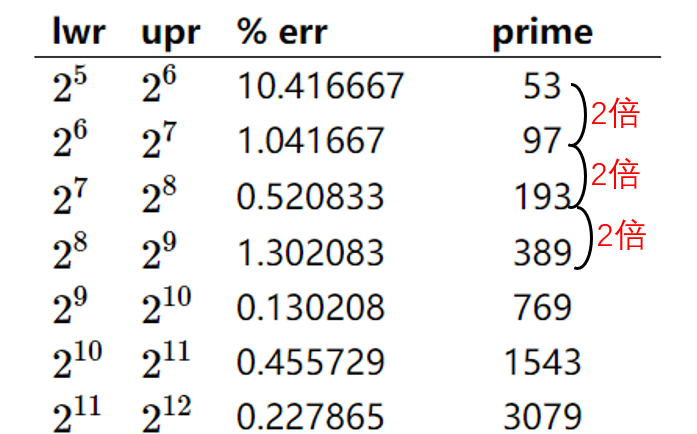对于原本元素个数为 upperTol * oldM = N 的元素增加到 upperTol * newM = 2 * N，才触发一次resize操作，相当于进行了翻倍操作。resize的时间复杂度为O(N)级别的，但是需要增加N个元素才触发一次resize操作，所以根据均摊时间复杂度，增删两种操作的时间复杂度为O(2)级别的复杂度，改查操作的时间复杂度为O(1)级别。

增加动态空间处理后，所有操作的时间复杂度均为O(1)级别。下面列一个详细的表格：

Copyright: 采用 知识共享署名4.0 国际许可协议进行许可